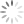Loading, please wait...## SQL Expressions

How To Use SQL Expressions

Introduction:

In this article, you will learn, how to use SQL expressions in SQL.

With the help of the expression, we find the data in SQL tables. Generally, it is the combination of the operators and symbols. We had written the expression, similar to the queries in our SQL Server. An expression normally considers the datatype of its data.

Syntax:

The basic syntax for Select Statement is as follows:

select column1, column2.....  from table_name  where [condition];

We have many types of SQL expressions. Some are as follows:

Boolean expressions in SQL:

SQL Boolean expressions obtains the similar values in the table.

The basic syntax for Boolean expression is as follows:

select column1, column2... from table_name where single value/ matching expression;

Here, we have an Employee table for showing the results.

select * from employee;

Step 1: We write the query for Boolean expressions.

Now, select and execute this query.

Step 2: The result is:

Numeric Expression in SQL:

SQL Numeric expression is used to implement the Arithmetic operations.

The basic syntax for the numeric expression is as follows:

select numerical_expression as  operation_name [from table_name where condition] ;

Step 1: We write the query for the numeric Expression.

Now, select and execute this query.

Step 2: The result is:

We include many numeric functions in this expression to calculate the data.

Step 1: We write the query for other numeric expression.

Now, select and execute this query.

Step 2: The result is:

Date expressions in SQL:

This expression gives the present Date and Time of System.

Syntax:

The basic syntax for date expressions is as follows:

select current_timestamp;

Step 1: We write the query for other numeric expression.

Now, select and execute this query.

Step 2: The result is:

Summary:

Thus, we learned that expressions helps to find the data in SQL with different ways.